4.1 A Phenomenological Trapping Model

The trapping dynamics in the simplest case are governed by the first-order rate equation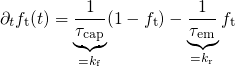(4.1)

with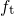being the electron occupancy of a single trap. Each of these traps is characterized by its own capture (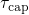) and emission (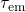) time constant, which are related to the forward (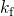) or the reverse (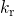) rate, respectively. The first term on the right-hand side of equation (4.1) has a positive sign, stands for electron trapping and increases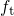. By contrast, detrapping is represented by the second term with the negative sign and causes a reduction in. It is emphasized that the defects have only discrete occupation numbers. This means that the defects carry an integer number of electrons and thusmust equal to either zero or unity. However, for a large number of defects,corresponds to the average occupation number and thus takes values within the range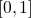. It is further mentioned that the physical trapping process is of a stochastic nature, as it has also been the case for electron tunneling and vibronic transitions in Chapter 2. These stochastic processes are characterized by the fact that the transition times are statistically distributed. As a consequence,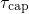and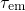correspond to the expectation value of the capture (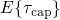) or emission (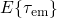) times, respectively, and the electron occupancy only changes gradually with time. The electron occupancy as a function of time is determined by the rate equation (4.1), which is a first-order differential equation with the solution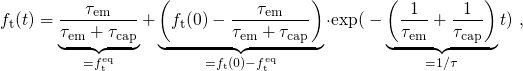(4.2)

and is depicted in Fig. 4.1. The first term of equation (4.2) corresponds to the equilibrium occupancy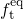, which approaches unity for the assumption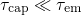. The second term describes an exponential transition of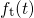towards. In a time-logarithmic representation this leads to a step of, occurring within three decades around. This step is associated with the expectation value of the transition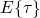in the trapping process and the stochastic nature of this process is reflected in the washed-out shape of the step. As demonstrated in Fig. 4.1, the step linearly shifts with the order of magnitude of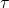. Furthermore, its height reaches the maximal value ofwhen the capture and emission time constant differ by some orders of magnitude. Note that hole detrapping requires that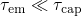and the time point of the transition is then determined by.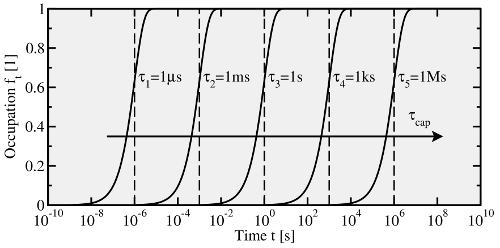Figure 4.1: The time evolution of the trap occupancy for five traps with their different trapping time constant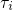assuming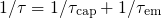and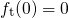.gives the time point, when the trapping process should occur according to its expectation value. In a time-logarithmic plot, the time point of the transition shifts linearly with the order of magnitude of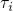.

One has to consider that the atomic structure of defects strongly influences the defect properties. Dielectric host materials, in particular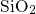and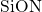, exhibit an amorphous structure with large variations in the bond lengths and angles, which are assumed to have an impact onand. As a result, the trapping time constants in equation (4.1) are subject to a dispersion. This will be accounted for by a probability density function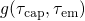, which is normalized by definition. Then the time evolution of trapped charges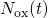can be expressed as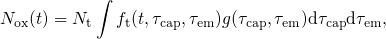(4.3)

where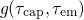is normalized and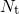stands for the concentration of traps. The integral in equation (4.3) can be interpreted as a superposition of individual and thus independent trapping processes. Note that this concept is in agreement with the findings of TDDS, in which the single trapping processes take place almost independently from each other.

In a phenomenological model of Yang et al. , the capture and emission time constants were correlated by the relation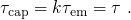(4.4)

Furthermore, Yang et al. assumed a log-normal distribution of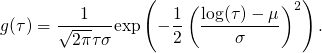(4.5)

Although his model allows reasonable fits to single relaxation curves, it is inconsistent with the scalability of the experimental data (see Section 1.4). This is due to the fact that neither the probability density function nor the time constants have a field- or temperature dependence, which were not considered. Nevertheless, the above formulation of the rates already includes the central aspects of a charge trapping model and thus has served as a foundation for other models. The following points are suggested as an improvement for Yang’s model:

• The probability density function has been phenomenologically introduced, where its shape was chosen to reproduce experimental data. Therefore, it is the goal to correlate the probability density function with certain physical properties of the defects and find reasonable shapes for.
• The model does not contain any information about the physics of the trapping process1. Therefore, expressions forandare sought, which are derived from physical considerations and must consequently depend on the temperature and the electrostatics in the device.